## SOWING SEEDS REVISITED

### SOLVING ONE AND TWO-STEP ALGEBRAIC EQUATIONS

Introduction to the relationship between partitive and quotative division to one-step algebraic equations.

## Intentionality & Unit Overview### Access each lesson from this unit using the navigation links below

Students will determine the number of green bean seeds planted per pot.

## Intentionality…

In this task, students will determine the total number of beans to sow per pot. In this context, the number of pots is known, and the quota per pot (the rate) is unknown. Students will likely fair share the total quantity of beans amongst the 7 pots in order to determine the number of beans in every pot. This context is partitive in nature. It can be represented by division. It can also be represented by multiplication with a variable in the place of the quota (or rate). Some of the big ideas that may emerge through this task include:

• There are two types of division;
Partitive division is when the total quota is known (the dividend), and the number of parts or groups (the divisor) is known;
• Partitive division reveals a rate;
• In partitive division, the dividend and the divisor often have different units;
• The dividend from any division sentence can be decomposed into smaller parts to allow for friendlier division by the divisor. This strategy is known as partial quotients. (i.e.: 85 ÷ 5 = 45 ÷ 5 + 40 ÷ 5 = 9 + 8 = 17);
• Division is the inverse operation of multiplication;
• Variables are used to represent changing or unknown quantities;
• When solving an equation where the coefficient of the unknown variable is not equal to 1, division is required to determine the value of the unknown variable and the context of the problem determines which type of division is required.

## What Do You Notice? What Do You Wonder?

Show students the following video:

What do you notice?

What do you wonder?Give students 60 seconds (or more) to do a rapid write on a piece of paper.

Replaying the video can be helpful here.

Then, ask students to share with their neighbours for another 60 seconds.
Finally, allow students to share with the entire group. Be sure to write down these noticings and wonderings on the blackboard/whiteboard, chart paper, or some other means to ensure students know that their voice is acknowledged and appreciated.

Some of the noticing and wondering that may come up includes:

• I noticed a package of green beans.
• I noticed there was information on the package.
• I noticed the beans were dumped out.
• I wonder how many beans were in the package.
• I wonder if there are any left in the package.
• I notice seven pots.
• And many more.

At this point, you can answer any wonders that you can cross off the list right away.

For example:

• Yes, this person is in fact planning to plant the seeds in small gardening pots.
• The package had quite a few seeds in it, but we aren’t sure how many there are.
• There are no beans left in the package. They were all dumped out.
• And so on.

## Estimation: Prompt

After we have heard students and demonstrated that we value their voice, we can land on the first question we will challenge them with:

How many beans were in the package?

Make an estimateWe can now ask students to make an estimate (not a guess) as we want them to be as strategic as they can possibly be. This will force them to determine a number of beans that would be reasonable before determining a more precise answer. Consider asking students to think about a number of beans that would be “too low” and a number that would be “too high” before asking for their best estimate in order to help them come up with a more reasonable estimate. Encourage students to share their estimates, however avoid sharing their justification just yet. We do not want to rob other students of their thinking.

## Estimation: Reveal

Show the following video: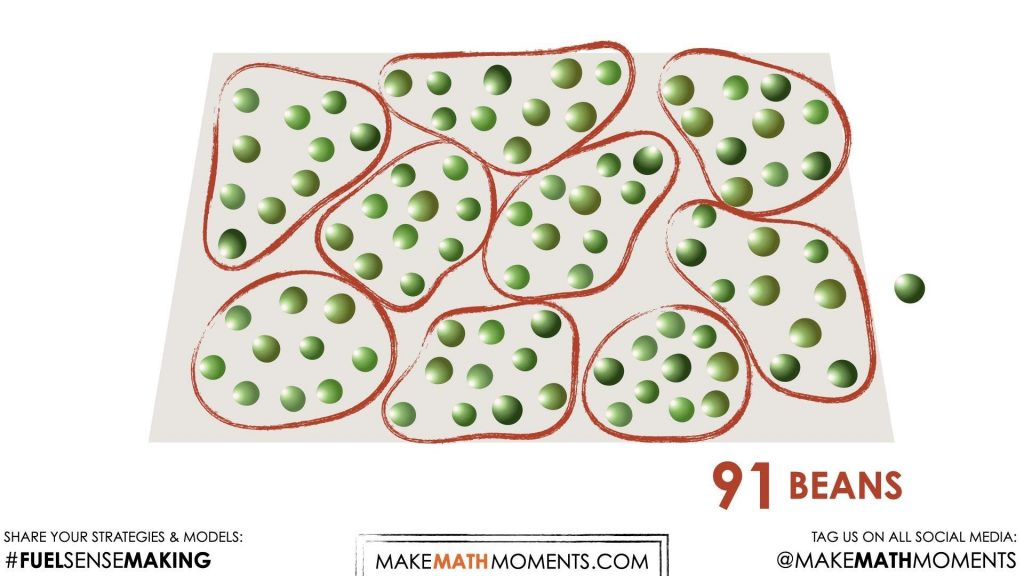Celebrate student estimates that were very close to the actual number of beans using a routine of your choice as we head into the sense making portion of this lesson.

## Crafting A Productive Struggle: Prompt

Now that we have confirmed that there are 91 beans to be planted, prompt students by stating:

All 91 beans were sown in seven pots. The gardener was careful to sow the same number of beans in every pot. How many beans did the gardener sow in each pot?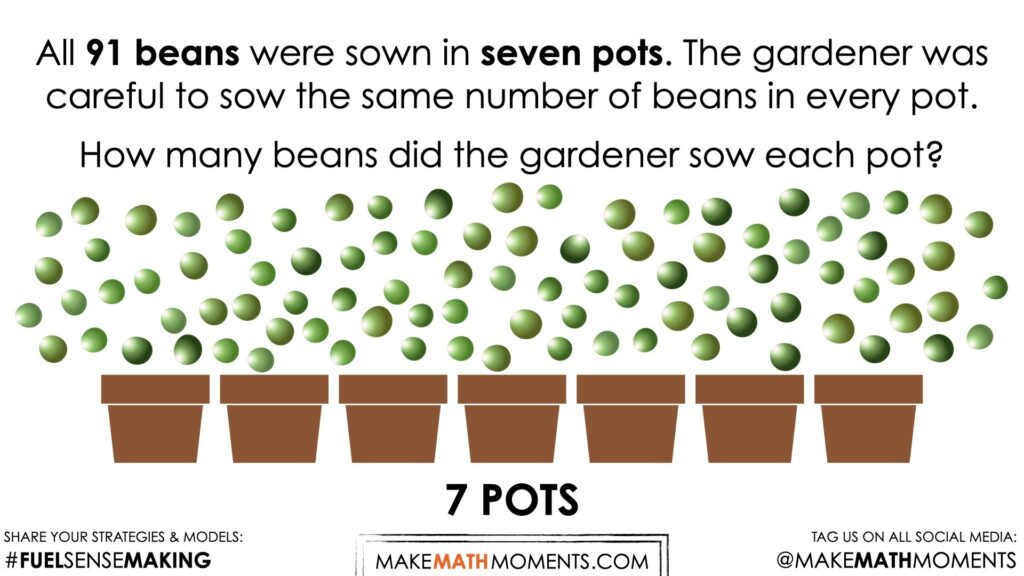Be sure to remind students that they are not to use a calculator to determine the number of beans per pot as using that tool will rob them of this mathematical experience. Students should use a mathematical model in order to communicate their thinking.

## While Students Are Productively Struggling…

Monitor student thinking by circulating around the room and listening to the mathematical discourse. Select and sequence some of the student solution strategies and ask a student from the selected groups to share with the class from:

• most accessible to least accessible solution strategies and representations;
• most common/frequent to least common/frequent strategies and representations; or,
• choose another approach to selecting and sequencing student work.

The tools and representations you might see students using to convince their peers and/or the teacher include:

• Concrete manipulatives to fairly distribute the beans
• An array through skip counting
An open-array using partial products
• A flexible algorithm using partial products

Have students share their strategies and reasoning for how to represent the number of beans per pot. Ask them to convince you and their peers that their answer is correct by sharing mathematical models.

Discuss their strategies and elicit student thinking during your consolidation to build off of their current prior knowledge and understanding rather than “fixing” or “funnelling” student thinking to a strategy and/or model that does not connect to their strategy and/or approach.

## Student Approach #1: Concrete Fair Sharing with Counting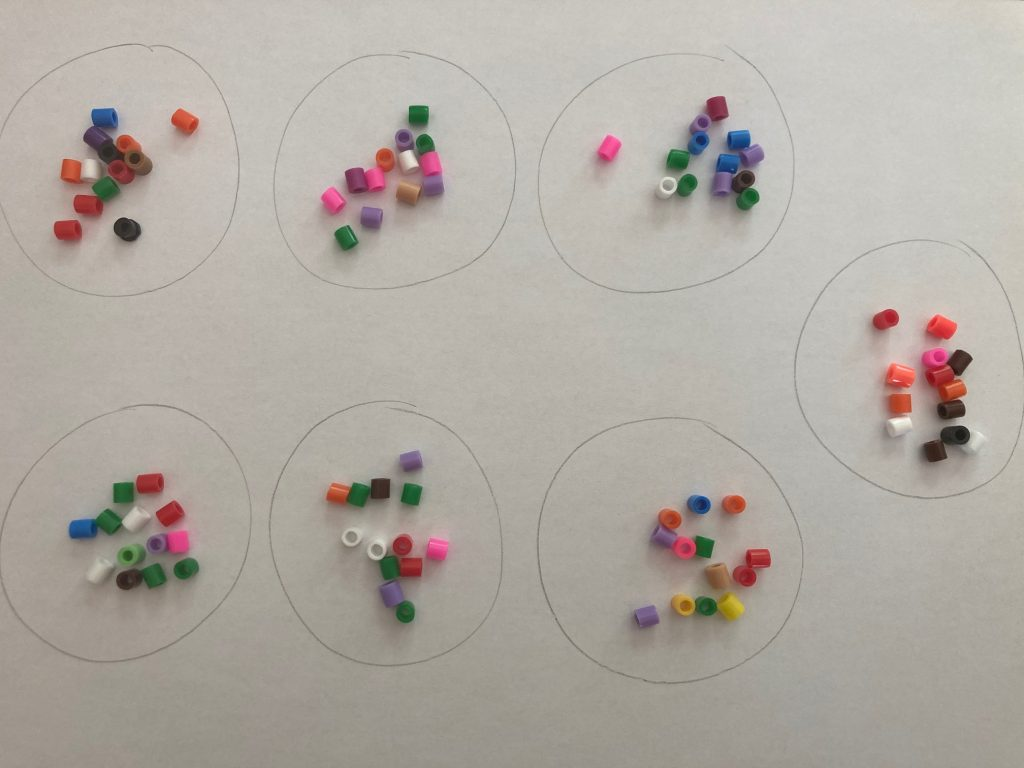I drew 7 circles to represent the pots. I knew that I had to share the beans between those pots. Putting one bean in at a time would take too long, so I started with ten beans. I counted by ten. I had 21 beans left. I added 2 beans to each pot. But I still had 7 beans leftover, that’s one more bean per pot. 13 beans altogether.

## Student Approach #2: Array Using Repeated Addition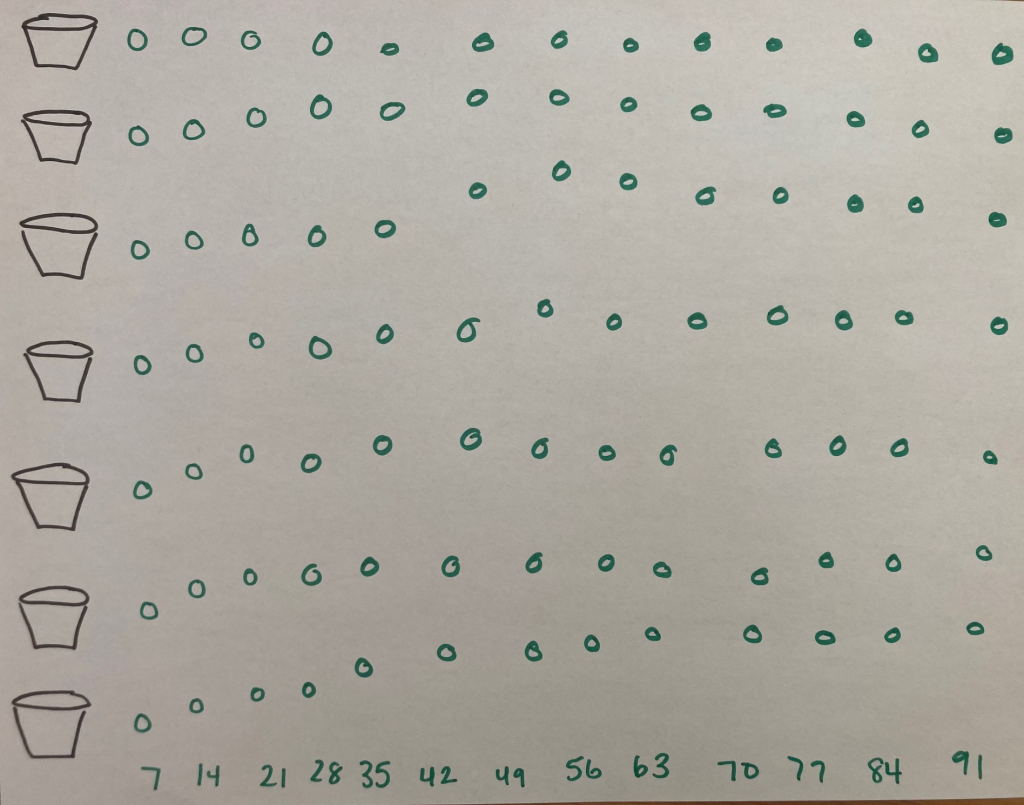I drew the seven pots along the side. I started by putting one pea in each pot. I kept doing this, adding 7 in each time, until I had all 91 beans. I needed to place 13 beans in each pot.

## Student Approach #3: Open-Array Using Partial-Products and Revealing Partial-Quotients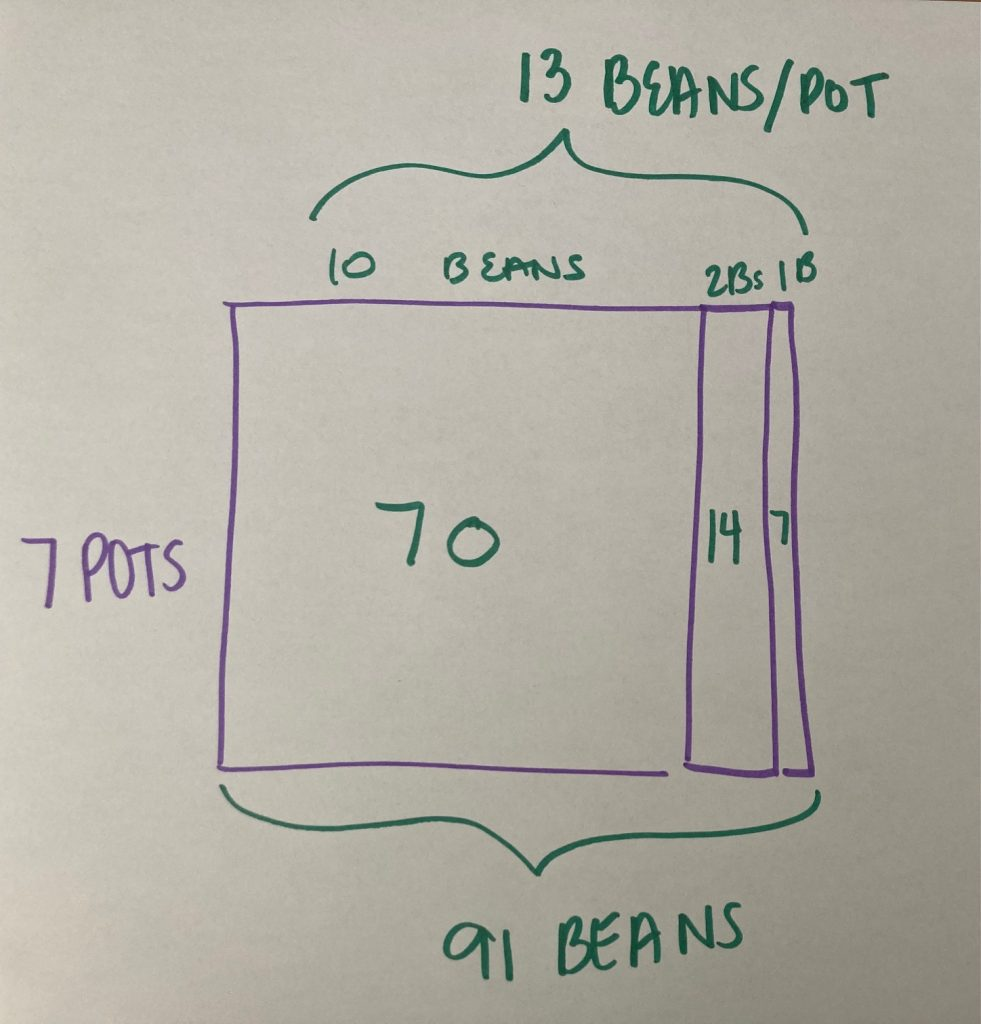I know that if I put 10 beans in every pot, that will use 70 of my total beans. I added two more to each pot, that’s 84 beans. So then I knew I had to add one more pea to every pot in order to use all of the 91 beans.

## Reveal

Show students the following reveal video:

Or consider sharing the final screenshot of the video: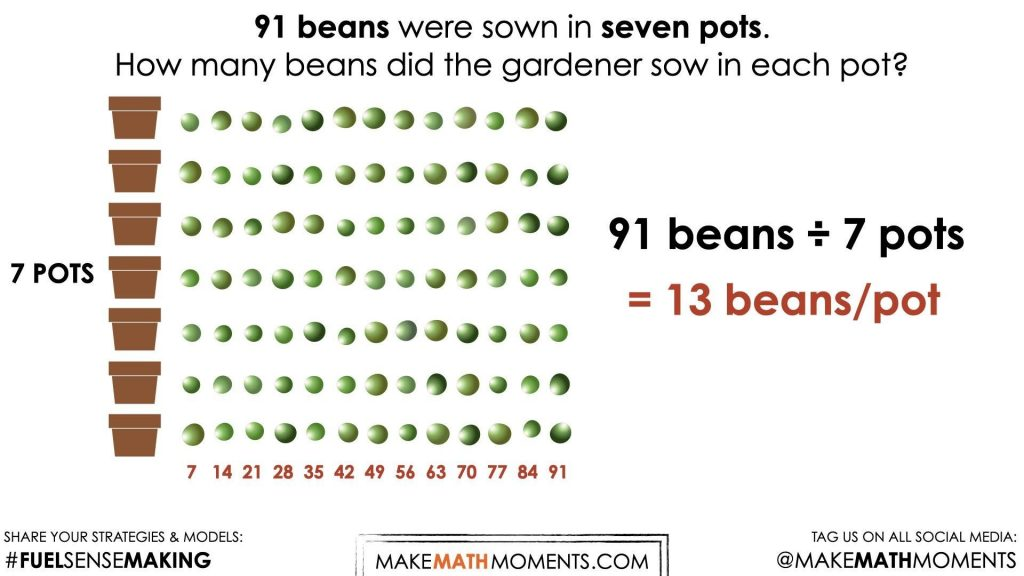## Consolidation

You might consider selecting student solutions that shift students from the set model (six circles and placing beans one at a time) towards an array model that promotes skip counting.

You can see this modelled below in the reveal video:The array, and eventually the open array, are powerful tools for solving division problems using the partial quotient strategy.

See this in the silent solution consolidation video below: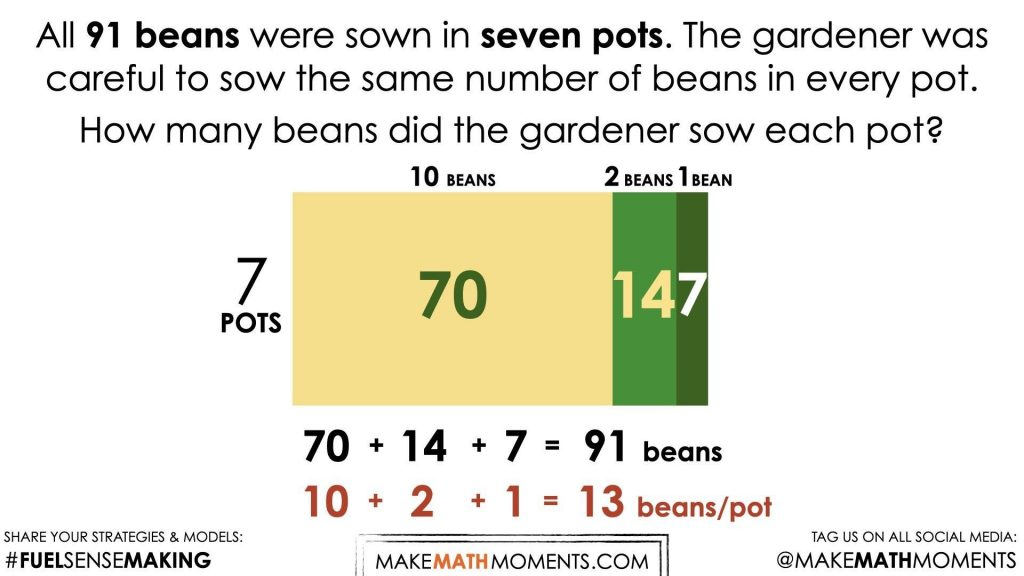At this point, it is a great idea to ask students the following prompt.

Prompt:

What operation did you use to solve this problem?

Give students the opportunity to turn and talk, and then share some of their thinking. Some students may say that they used counting, addition, subtraction, multiplication or division depending on their strategy.

At this point, students may or may not have realized that this context can be represented by a division sentence. This is your opportunity to name this behavior as division. In particular, partitive division.

91 beans ÷ 7 pots = 13 beans/pot

However, this context can also be represented by the following equation.

7(r) = 91

Give students an opportunity to discuss the equation. How is it the same, how is it different from the division shown above?

Why is there a variable, what does r represent?

Encourage students to name the units in this equation.

7 pots(unknown number of beans per pot) = 91 beans

In this context, the variable is represented by r because it is a rate.

This is a one-step algebraic equation, because it only requires one step to determine the value of the unknown quantity. The unknown number of beans per pot can be revealed through partitive division, the inverse operation to multiplication.

Facilitator Note:
If students are unfamiliar with the use of brackets to represent multiplication, consider using the operator more explicitly and moving towards the use of brackets.

## Reflect and Consolidation Prompts

Provide students an opportunity to reflect on their learning by offering this consolidation prompt to be completed independently.

### Consolidation Prompt #1:

Division is the inverse operation of multiplication. Defend this statement.

### Consolidation Prompt #2:

Consider the equation below. Write a context that could be represented by this equation. Model and solve.

8(b) = 48

We suggest collecting this reflection as an additional opportunity to engage in the formative assessment process to inform next steps for individual students as well as how the whole class will proceed.

## Educator Discussion Area

Login/Join to access the entire Teacher Guide, downloadable slide decks and printable handouts for this lesson and all problem based units.

## Explore Our 60+ Problem Based Units

This Make Math Moments Lesson was designed to spark curiosity for a multi-day unit of study with built in purposeful practice, number talks and extensions to elicit and emerge strategies and mathematical models.

Dig into our other units of study and view by concept continuum, grade or topic!